﻿ 平板喷气粘性流场数值计算方法研究
 舰船科学技术2016, Vol. 38Issue (8): 47-51PDF

Numerical study of method of flat plate viscous flow field with bubble
WU Hao, OU Yong-peng
Department of Naval Architecture Engineering, Naval University of Engineering, Wuhan 430033, China
Abstract: In order to investigate the complexity of gas-liquid mixing under different condition of flat plate, a method with the combination of RANS equations and Mixture model is proposed for the viscous-flow calculation of a large flat bottom ship. There are eight different numerical calculation method formed by a combination 4 kinds of turbulence models, grid, and 3 kinds of wall treatment. The influence of wall function, the first layer of mesh wall and turbulence models for numerical results was analyzed. Experimental results were compared with the numerical study. The results show that: RNG k-ε turbulence model, standard wall function, 1mm first layer of mesh, y + for the calculation of 31 to 35, the results can be used for mixed-flow analysis.
Key words: gas-liquid mixing     Mixture model     wall function     turbulence model
0 引言

1 Mixture两相流模型

Mixture模型的控制方程由连续性方程和动量方程构成，其具体表达形式如下：

 $\frac{\partial }{{\partial t}}({\rho _m}) + \nabla \cdot ({\rho _m}{\vec v_m}) = 0 \text{，}$ (1)

 ${\vec v_m} = \frac{{\sum\limits_{k = 1}^n {{\alpha _k}{\rho _k}{{\vec v}_k}} }}{{{\rho _m}}} \text{。}$ (2)

 \begin{aligned} \frac{\partial }{{\partial t}}({\rho _m}{{\vec v}_m}) \!+\! \nabla \! \cdot \!({\rho _m}{{\vec v}_m}{{\vec v}_m}) \!=\! -\nabla p \!+\! \nabla \cdot [{\mu _m}(\nabla {{\vec v}_m} \!+\! \nabla \vec v_m^T)] + \\ \quad \quad \quad \quad \quad {\rho _m}\vec g + \vec F + \nabla \cdot (\sum\limits_{k = 1}^n {{\alpha _k}{\rho _k}} {{\vec v}_{dr,k}}{{\vec v}_{dr,k}}) \text{。} \\ \end{aligned} (3)

 ${\mu _m} = \sum\limits_{k = 1}^n {{\alpha _k}{\mu _k}} \text{，}$ (4)
 ${v_{dr,k}} = {\vec v_k}-{\vec v_m} \text{。}$ (5)

 ${\vec v_{pq}} = \frac{{{\tau _p}}}{{{f_{drag}}}} \cdot \frac{{({\rho _p}-{\rho _m})}}{{{\rho _p}}}\vec a \text{，}$ (6)
 ${\tau _p} = \frac{{{\rho _p}d_p^2}}{{18{\mu _q}}} \text{。}$ (7)

 $\vec a = \vec g-({\vec v_m} \cdot \nabla ){\vec v_m}-\frac{{\partial {{\vec v}_m}}}{{\partial t}} \text{。}$ (8)

 ${f_{drag}} = \left\{ \begin{gathered} 1 + 0.15{{Re} ^{0.687}} \text{，} \quad {Re} \leqslant 1000 \text{，} \\ 0.0183{Re} \text{，} \quad \quad \;\;\;{Re} > 1000 \text{。} \\ \end{gathered} \right.\quad \;$ (9)
2 湍流模型及壁面处理方法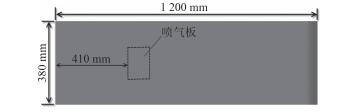图 1 计算平板主尺度示意图 Fig. 1 The main parameters of plate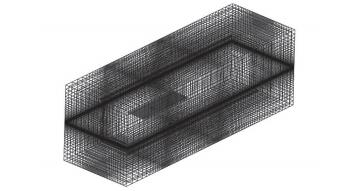图 2 平板流场区域及网格布局 Fig. 2 The flow field of plate and its mesh distribution

1）标准壁面函数法。y+应大于30～60，最好接近30，湍流边界层内应布置一定的网格数量。标准壁面函数不适用于层流底层，因此第1层网格应布置在对数律层内。

2）增强壁面函数法。该方法可用于计算层流底层的流动，当用于计算层流底层时，y+最好取值为1左右，但如果第1层网格布置在了层流层内，略高的y+值也可行。计算过程中，层流底层内应布置至少10个网格节点。

3）对于S-A模型（Spalart-Allmaras），若采用增强壁面函数，则y+=1；若采用标准壁面函数，则y+＞30。

4）对于低雷诺数k-ω模型，网格要求与增强壁面函数相同；采用高雷诺数k-ε模型时，网格要求与标准壁面函数相同。

5）对于LES模型，壁面附近的网格要求与增强壁面函数的要求相同。表 1 湍流模型及壁面函数 Tab.1 The turbulence models and wall functions
3 气层形态分析 3.1 气层宏观形态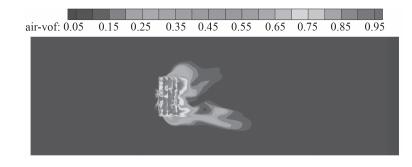图 3 计算方案1（S-A模型，y+=3） Fig. 3 The first calculation case by S-A turbulence model at y+=3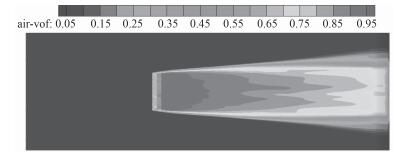图 4 计算方案2（S-A模型，y+=10） Fig. 4 The second calculation case by turbulence S-A model at y+=10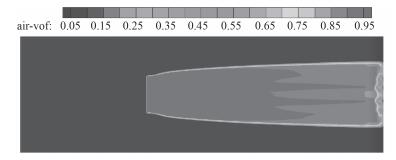图 5 计算方案3（k-ω模型） Fig. 5 The third calculation case by k-ω model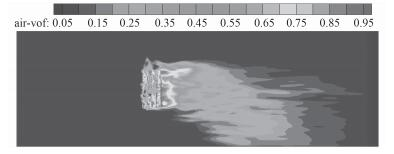图 6 计算方案4（LES模型） Fig. 6 The fourth calculation case by LES model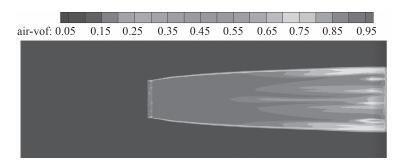图 7 计算方案5（RNG k-ε模型及增强壁面函数，y+=2） Fig. 7 The fifth calculation case by RNG k-ε model and enhance wall function at y+=2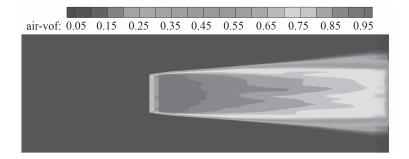图 8 计算方案6（RNG k-ε模型及增强壁面函数，y+=10） Fig. 8 The sixth calculation case by RNG k-ε model and enhance wall function at y+=10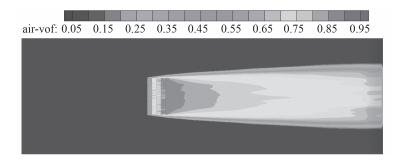图 9 计算方案7（RNG k-ε模型及增强壁面函数，y+=20） Fig. 9 The seventh calculation case by RNG k-ε model and enhance wall function at y+=20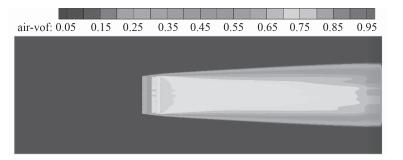图 10 计算方案8（RNG k-ε模型及标准壁面函数，y+=30） Fig. 10 The eighth calculation case by RNG k-ε model and standard wall function at y+=30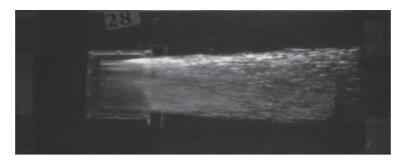图 11 平板底部气层模型试验照片 Fig. 11 The air layer photo under plate of test result

3.2 气层厚度及宽度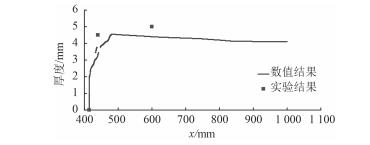图 12 V=1.287 m/s，Q=1.08 m3/h气层厚度沿平板长度方向变化的计算值与实验结果 Fig. 12 The air layer thickness of numerical results and test result at V=1.287 m/s and Q=1.08 m3/h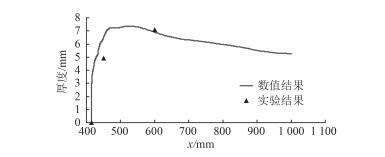图 13 V=0.838 m/s，Q=1.11 m3/h气层厚度沿平板长度方向变化的计算值与实验结果 Fig. 13 The air layer thickness of numerical result and test result at V=0.838 m/s and Q=1.11 m3/h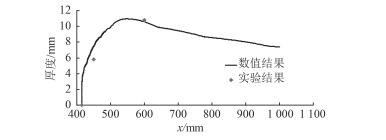图 14 V=1.287 m/s，Q=2.061 m3/h气层厚度沿平板长度方向变化的计算值与实验结果 Fig. 14 The air layer thickness of numerical result and test result at V=1.287 m/s and Q=2.06 m3/h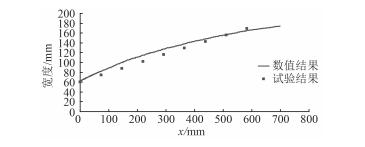图 15 V=1.287 m/s，Q=2.061 m3/h沿平板长度方向气层厚度的计算值与实验结果 Fig. 15 The air layer thickness of numerical result and test result at V=1.287 m/s and Q=2.06 m3/h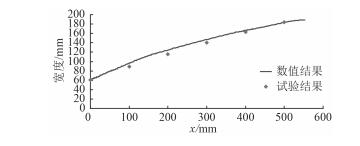图 16 V=0.837 m/s，Q=1.04 m3/h沿平板长度方向气层厚度的计算值与实验结果 Fig. 16 The air layer thickness of numerical result and test result at V=0.837 m/s and Q=1.04 m3/h

4 结语

1）采用RNG k-ε湍流模型、标准壁面函数、第1层网格1 mm、y +为31～35的计算方案可较好地模拟平板底部气液混合层的宏观形态。

2）采用Mixture模型与RNG k-ε湍流模型相结合的方法可有效模拟气层沿平板宽度方向的扩散边界和气层厚度沿流动方向的变化规律，说明搞方法对模拟气液混合流气层形态有效。

  CHOI J K, HSIAO C T, CHAHINE G L. Numerical studies on the hydrodynamic performance and the startup stability of high speed ship hulls with air plenums and air tunnels[C]//Proceedings of the Ninth International Conference on Fast Sea Transportation FAST2007. Shanghai, China, 2007: 476-484.  KIM D, MOIN P. Direct numerical Simulation of air layer drag reduction over a backward-facing step[C]//Proceedings of the 63rd annual Meeting of the APS Division of Fluid Dynamics. Long Beach, California: American Physical Society, 2010: 351-363.  LIEM H C, TODA Y, SANADA Y. A consideration on drag reduction by air lubrication using integral type boundary layer computation[J]. Journal of the Japan Society of Naval Architects and Ocean Engineers , 2011, 13 :59–65. DOI:10.2534/jjasnaoe.13.59  LI Y B, WU X Y, MA Y, et al. A method based on potential theory for calculating air cavity formation of an air cavity resistance reduction ship[J]. Journal of Marine Science and Application , 2008, 7 (2) :98–101. DOI:10.1007/s11804-008-7077-x  吴晓宇.气泡船大尺寸多凹槽稳定气穴形成理论研究[D].哈尔滨:哈尔滨工程大学, 2008. http://cdmd.cnki.com.cn/article/cdmd-10217-2009060258.htm  董文才, 郭日修, 朱凤荣, 等. 平板湍流边界层内气泡流流动实验研究[J]. 海军工程大学学报 , 2001, 13 (3) :34–37.  郑晓伟, 王家楣, 曹春燕. 二维船舶微气泡减阻数值模拟[J]. 船舶工程 , 2005, 27 (6) :15–18.  蔡红玲.高速气泡船流场数值模拟[D].武汉:武汉理工大学, 2008.  杨鹏.气泡船三维粘性绕流的数值模拟[D].武汉:武汉理工大学, 2008. http://cdmd.cnki.com.cn/article/cdmd-10497-2008109663.htm  曹春燕.船舶微气泡减阻数值模拟[D].武汉:武汉理工大学, 2003. http://cdmd.cnki.com.cn/article/cdmd-10497-2003095411.htm  荒賀浩一, 松井良輔, 脇本辰郎, 等. 界面活性剤水溶液の水平円管内流れに及ぼす微細気泡の影響[J]. 実験力学 , 2010, 10 (3) :304–311.  梁志勇, 陈池. 微气泡对平板摩擦阻力影响的分析[J]. 上海大学学报(自然科学版) , 2002, 8 (3) :267–272.  FELTON K, LOTH E. Diffusion of spherical bubbles in a turbulent boundary layer[J]. International Journal of Multiphase Flow , 2002, 28 (1) :69–92. DOI:10.1016/S0301-9322(01)00060-X  郭峰, 欧勇鹏, 董文才, 等. 平板微气泡减阻预报及影响因素研究[J]. 中国造船 , 2008, 49 (S) :66–74.  MORIGUCHI Y, KATO H. Influence of microbubble diameter and distribution on frictional resistance reduction[J]. Journal of Marine Science and Technology , 2002, 7 (2) :79–85. DOI:10.1007/s007730200015